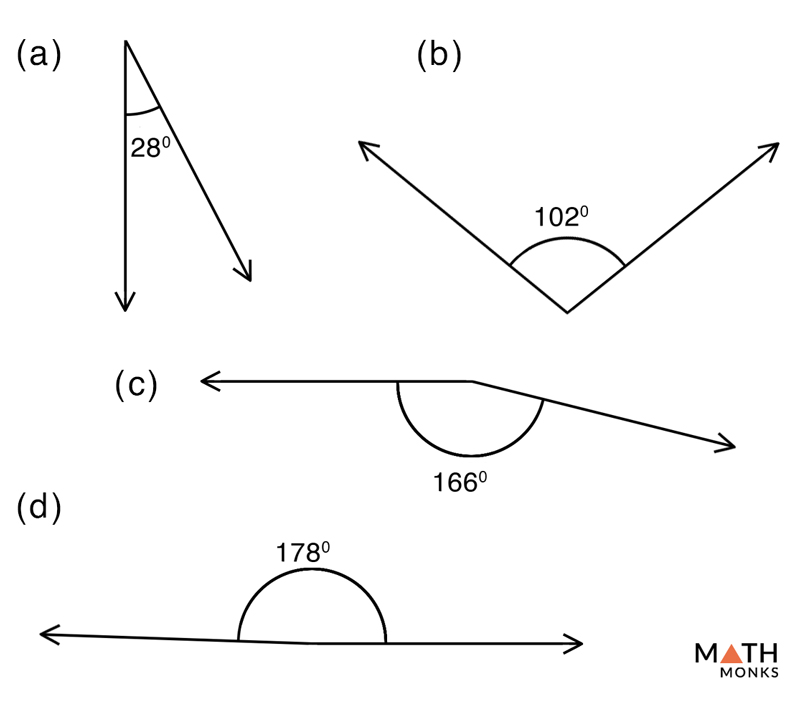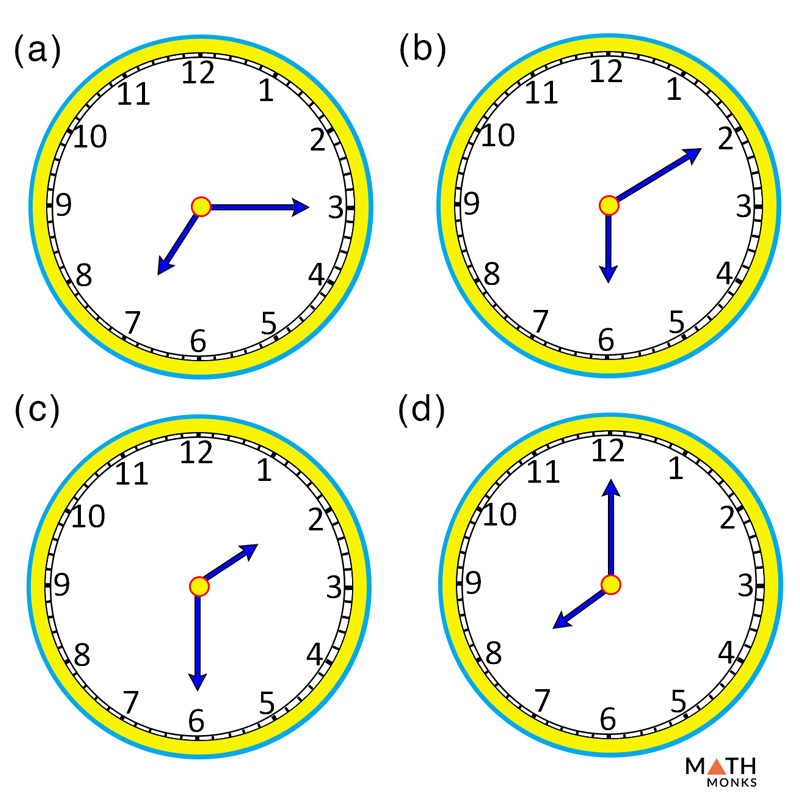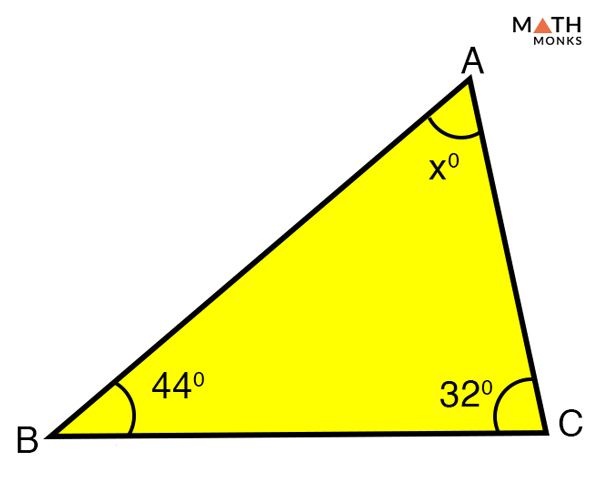# Obtuse Angle

## What is an Obtuse Angle

An obtuse angle is defined as an angle that measures more than 90° and less than 180°. In other words, any angle that lies between 90° and 180° is an obtuse angle.

A few more examples of obtuse angle are shown below:

## Obtuse Angles in Real Life

• One angle in an obtuse angle triangle
• All angles of a regular polygon with five or more sides
• Blades of a ceiling fan
• A coat hanger
• Hands of a wall clock showing 8’ o clock
• An elevation or a staircase
• A house ceiling
• A fully opened cupboard door
• The angle at the bottom of the pot that holds a plant
• The angle at the bottom of some coffee mug
• A book wide open
• Most corners of our roomChoose from the given options the obtuse angles.

Solution:

As we know,
Obtuse angles are angles measuring more than 90° and less than 180°,
Hence, options (b), (c), and (d) are obtuse angles.Which of the following options given in the clock timing represents an obtuse angle?

Solution:

As we know,
Obtuse angles are angles measuring more than 90° and less than 180°,
Hence, options (a), (b), (c), and (d) are obtuse angles.Find x in the given triangle and state whether it is an obtuse angle triangle

Solution:

As we know,
The sum of the interior angles in a triangle = 180°,
Thus,
In △ABC,
∠ABC + ∠BCA + ∠CAB = 180°, here ∠ABC = 44°, ∠BCA = 32°
44° + 32° + ∠CAB = 180°
∠CAB = 180° – (44° + 32°)
∠CAB = (x°) = 104°
Since ∠CAB measure more than 90° and less than 180°, △ABC is an obtuse angle triangleIdentify the obtuse angles in the given polygon ABCDEF.

Solution:

As we know,
The sum of the interior angles of a hexagon = 720°
Thus,
∠ABC +∠BCD + ∠CDE + ∠DEF + ∠EFA +∠FAB = 720°
132° + 82° + ∠CDE + 88° + 107° + 142° = 720°
∠CDE = 720° – 551°
∠CDE = (x°) = 169°
Thus ∠ABC, ∠EFA, and ∠FAB are obtuse angles.

## Acute vs Obtuse Angle

The main difference between an acute and an obtuse angle is that an acute angle always measures less than 90°, whereas an obtuse angle measure more than 90° and less than 180°.

## FAQs

Q1.How many obtuse angles are in an obtuse triangle?

Ans. There is only one obtuse angle in an obtuse triangle.

Q2. Can a triangle have two obtuse angles?

Ans. No, a triangle cannot have two obtuse angles because the sum of the three angles in a triangle is always 180°. If there are two obtuse angles that measure more than 90°, the third angle will have a negative value, which is impossible.

Q3. Can a quadrilateral have 4 obtuse angles?

Ans. No, a quadrilateral cannot have four obtuse angles.

Q4. Can a right triangle have an obtuse angle?

Ans. No, a right triangle cannot have any obtuse angles.

Q5. Can a trapezoid have four obtuse angles?

Ans. A trapezoid cannot have four obtuse interior angles.

Q6. What do the bisector of an obtuse angle forms?

Ans. The bisector of an obtuse angle forms two acute angles that have the same measure.